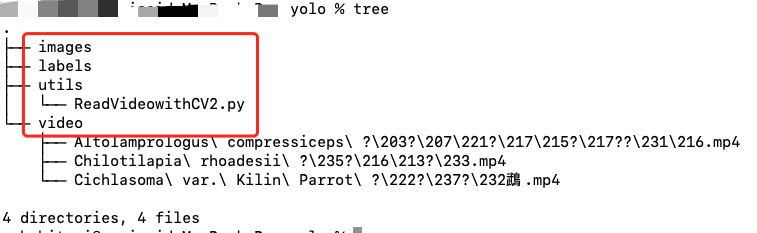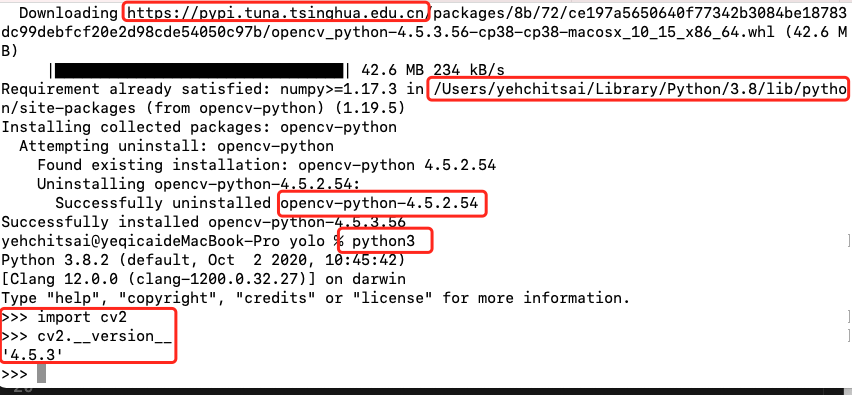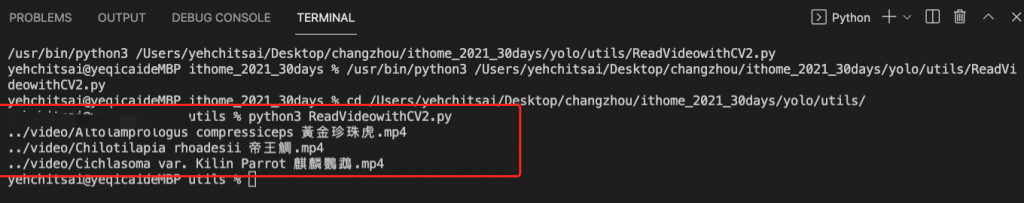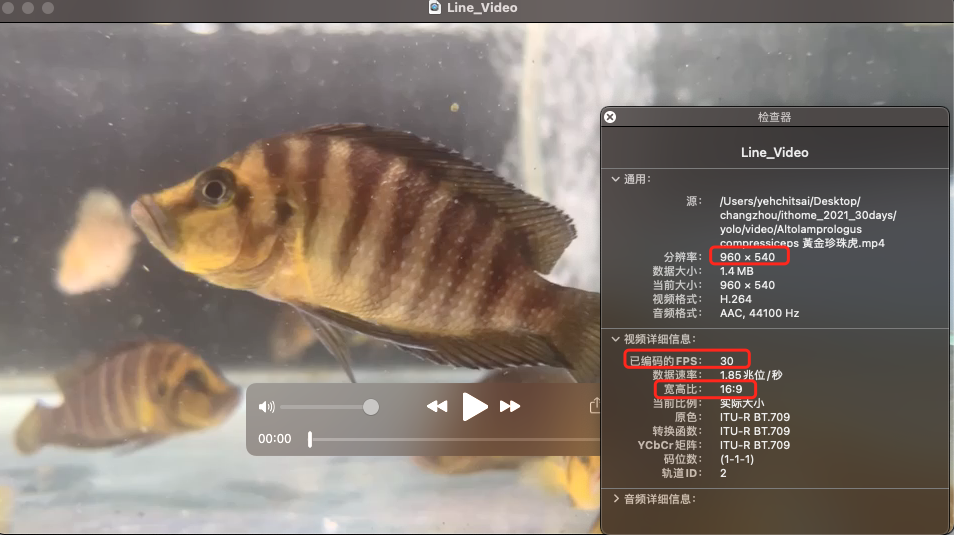DAY 11
1
AI & Data

# 將影片轉換成圖片 － Day 11

1. 建立目錄結構
2. 安裝需要的套件
3. 撰寫程式
1. 讀寫目錄
2. 影片轉圖片
3. 變更尺寸為608

github 上所下載的影片放在 video 文件夾，而 utils 文件夾放資料預處理的程式，如今天需要用到的將影片轉成圖片，images 用來放處理後的圖片，labels 是用來存放標籤文件，如下圖所示。``````pip3 install opencv-python
python3
``````

``````import cv2
cv2.__version__
import sys
sys.path
``````1. 讀寫目錄：指定輸入 (video) 與輸出目錄 (images)
2. 影片轉圖片： 使用 openCV 進行轉換，因為拍攝時時每秒 30 張，但這樣的圖片變化太少，所以是每 5 張圖片取一張。
3. 變更尺寸： YOLOV3 可以自行在 cfg 檔中設定輸入圖片的尺寸，預設 416×416 、608×608 或任何大於 32 的 2 次方倍數尺寸，再尺寸的縮放上還需要注意的是長寬比，因為大多數的照片的是矩型，但 YOLOV3 接受的卻是正方形，所以必須要針對長寬不足的地方進行處理，我們這邊是直接填 0 ，也就是黑色。
``````import cv2
import glob
import os

# 變更到指定尺寸，長寬邊不足者補黑色
def process_image(img, min_side = 608):
size = img.shape
h, w = size, size
scale = max(w, h) / float(min_side)
new_w, new_h = int(w/scale), int(h/scale)
resize_img = cv2.resize(img, (new_w, new_h),cv2.INTER_AREA) # 變更尺寸
if new_w % 2 != 0 and new_h % 2 == 0:
top, bottom, left, right = (min_side-new_h)//2, (min_side-new_h)//2, (min_side-new_w)//2 + 1, (min_side-new_w)//2
elif new_h % 2 != 0 and new_w % 2 == 0:
top, bottom, left, right = (min_side-new_h)//2 + 1, (min_side-new_h)//2, (min_side-new_w)//2, (min_side-new_w)//2
elif new_h % 2 == 0 and new_w % 2 == 0:
top, bottom, left, right = (min_side-new_h)//2, (min_side-new_h)//2, (min_side-new_w)//2, (min_side-new_w)//2
else:
top, bottom, left, right = (min_side-new_h)//2 + 1, (min_side-new_h)//2, (min_side-new_w)//2 + 1, (min_side-new_w)//2
pad_img = cv2.copyMakeBorder(resize_img, top, bottom, left, right, cv2.BORDER_CONSTANT, value=[0,0,0])

# 讀寫目錄
inputPath = '../video'
outputPath = '../images'

files = os.path.join(inputPath,'*.mp4')
files_grabbed = []
files_grabbed.extend(sorted(glob.iglob(files)))

for videoId in range(len(files_grabbed)):
print(files_grabbed[videoId])
raw = cv2.VideoCapture(files_grabbed[videoId])
fIndex = 1
fCount = 0

while 1:
# 影片轉圖片
fCount += 1
if (ret == True) :
if (fCount % 5) == 0:
img_pad = process_image(frame, min_side = 608)
fIndex += 1
else:
break
``````0